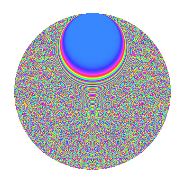# Properties

 Label 3150.2.eoLevel 3150 Weight 2 Character orbit eo Rep. character $$\chi_{3150}(317,\cdot)$$ Character field $$\Q(\zeta_{60})$$ Dimension 3840 Sturm bound 1440

# Related objects

## Defining parameters

 Level: $$N$$ = $$3150 = 2 \cdot 3^{2} \cdot 5^{2} \cdot 7$$ Weight: $$k$$ = $$2$$ Character orbit: $$[\chi]$$ = 3150.eo (of order $$60$$ and degree $$16$$) Character conductor: $$\operatorname{cond}(\chi)$$ = $$1575$$ Character field: $$\Q(\zeta_{60})$$ Sturm bound: $$1440$$

## Dimensions

The following table gives the dimensions of various subspaces of $$M_{2}(3150, [\chi])$$.

Total New Old
Modular forms 11648 3840 7808
Cusp forms 11392 3840 7552
Eisenstein series 256 0 256

## Trace form

 $$3840q + O(q^{10})$$ $$3840q - 12q^{15} - 480q^{16} - 36q^{17} - 16q^{18} - 36q^{27} + 12q^{30} + 20q^{33} + 40q^{39} + 4q^{42} + 140q^{45} + 48q^{50} - 32q^{57} - 24q^{58} - 8q^{60} + 228q^{63} - 24q^{65} + 140q^{69} - 8q^{72} - 232q^{75} + 48q^{77} + 16q^{78} + 120q^{84} - 40q^{87} + 300q^{89} + 80q^{90} + 24q^{92} + 12q^{93} + O(q^{100})$$

## Decomposition of $$S_{2}^{\mathrm{new}}(3150, [\chi])$$ into newform subspaces

The newforms in this space have not yet been added to the LMFDB.

## Decomposition of $$S_{2}^{\mathrm{old}}(3150, [\chi])$$ into lower level spaces

$$S_{2}^{\mathrm{old}}(3150, [\chi]) \cong$$ $$S_{2}^{\mathrm{new}}(1575, [\chi])$$$$^{\oplus 2}$$

## Hecke Characteristic Polynomials

There are no characteristic polynomials of Hecke operators in the database